Next: Wave packets and wave Up: Solution of Schrodinger equation Previous: Solution of Schrodinger equation

##### Problems

Problem 1:

The ground state wavefunction for a particle in a one-dimensional box of length L is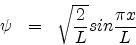Suppose the box is 10.0nm long. Calculate the probability that the particle is between x=4.95 nm and 5.05 nm.

Solution:

The probability of finding the particle between x and x + dx is given by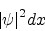. Therefore the required probability is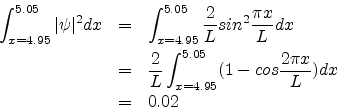Problem 2:

The ground state wave function of the hydrogen atom is given by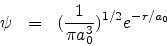a0 = 0.53A0 is the Bohr radius. Calculate the probability that the electron would be found somewhere within a small sphere of radius 1pm centred at the nucleus.

Solution:

The required probability is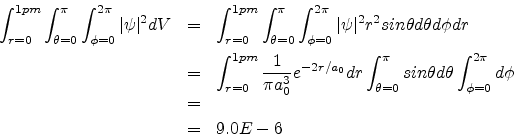Abhijit Poddar
2007-09-27